Courses

# Turbulent Flow In Pipes

## 9 Questions MCQ Test Mock Test Series for Civil Engineering (CE) GATE 2020 | Turbulent Flow In Pipes

Description
This mock test of Turbulent Flow In Pipes for Civil Engineering (CE) helps you for every Civil Engineering (CE) entrance exam. This contains 9 Multiple Choice Questions for Civil Engineering (CE) Turbulent Flow In Pipes (mcq) to study with solutions a complete question bank. The solved questions answers in this Turbulent Flow In Pipes quiz give you a good mix of easy questions and tough questions. Civil Engineering (CE) students definitely take this Turbulent Flow In Pipes exercise for a better result in the exam. You can find other Turbulent Flow In Pipes extra questions, long questions & short questions for Civil Engineering (CE) on EduRev as well by searching above.
QUESTION: 1

Solution:
QUESTION: 2

### In a turbulent flow in a pipe, the shear stress is

Solution:

In turbulent flow shear stress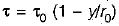where,
y = Distance measured from pipe wall
ζ0 = Shear stress at boundary

QUESTION: 3

### In the case of turbulent flows in circular pipes, the maximum velocity um and the mean velocity V are related as (um - V)lu =

Solution:

Turbulent flow
For smooth pipe,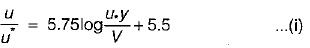Average velocity,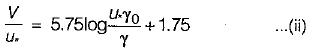y = distance measured from pipe boundary
u = shear velocity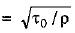from equation (i)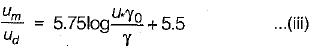(iii) - (ii) gives
(b) Rough pipe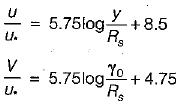It gives,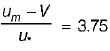same for both pipes rough or smooth

QUESTION: 4

The distance from pipe boundary, at which the turbulent shear stress is one-third the wall shear stress, is

Solution:

In turbulent flow shear stress at distance y from pipe boundary,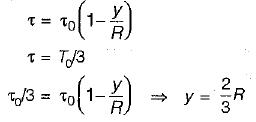QUESTION: 5

A flow in which the quantity of liquid flowing per second is not constant, is called

Solution:

A fluid motion is said to be turbulent when the fluid particles move in an entirely haphazard or disorderly manner, that results in a rapid and continuous mixing of the fluid leading to momentum transfer as flow occurs. At any point in a turbulent flow the velocity and pressure are the functions of time thereby rendering such a flow as unsteady.

QUESTION: 6

Which of following conditions would entail a greater energy dissipation in turbulent flow?
1. Smaller eddy size
2. Lower viscosity
3. Large intensity ofturbulence

Select the correct answer using the codes given below:

Solution:
QUESTION: 7

Match List-I (Nature of flow) with List-II (Friction factor equation) and select the correct answer using the codes given below the lists:
List- I
1.Turbulent flow with rough boundary
2. Turbulent flow with smooth boundary
3. Turbulent flow with Re < 105
4. Laminar flow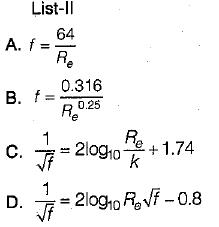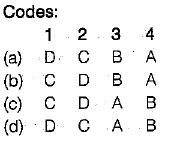Solution:
QUESTION: 8

Turbulent flow generally occurs

Solution:

Reynolds number,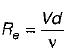When Re < Rec Laminar flow occurs at very small velocities or through narrow passage (small d) or for highly viscous fluids (high v) the Re will be small and flow will remain laminar. For high velocities through large passage Reynolds number will be sufficient to cause turbulence.

QUESTION: 9

A pipe line carrying water has surface-protrusions of average height 0.10 mm. If the shear stress developed is 7.85 N/m2, then the pipe surface will act as

Solution: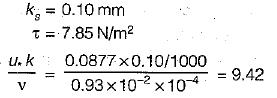Since roughness value lies between 4 and 100 the pipe act as in transition.